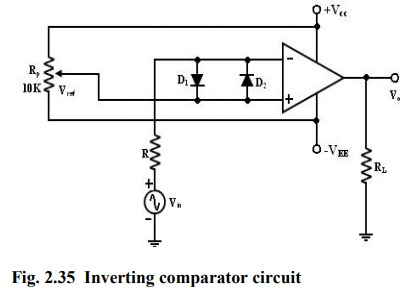Home | | Linear Integrated Circuits | Comparator using Operational Amplifier

# Comparator using Operational Amplifier

A comparator compares a signal voltage on one input of an op-amp with a known voltage called a reference voltage on the other input.

Comparator

A comparator compares a signal voltage on one input of an op-amp with a known voltage called a reference voltage on the other input. Comparators are used in circuits such as,

·           Digital Interfacing

·           Schmitt Trigger

·           Discriminator

·           Voltage level detector and oscillators

## Non-inverting Comparator:A fixed reference voltage Vref of 1 V is applied to the negative terminal and time varying signal voltage Vin is applied to the positive terminal.

When Vin is less than Vref the output becomes V0 at –Vsat

[Vin < Vref => V0 (-Vsat)].

When Vin is greater than Vref, the (+) input becomes positive, the V0 goes to +Vsat.

[Vin > Vref => V0 (+Vsat)].

Thus the V0 changes from one saturation level to another.

The diodes D1 and D2 protect the op-amp from damage due to the excessive input voltage Vin. Because of these diodes, the difference input voltage Vid of the op-amp diodes are called clamp diodes.

The resistance R in series with Vin is used to limit the current through D1 and D2. To reduce offset problems, a resistance Rcomp = R is connected between the (-ve) input and Vref.## Inverting Comparator:

This fig shows an inverting comparator in which the reference voltage Vref is applied to the (+) input terminal and Vin is applied to the (-) input terminal.In this circuit Vref is obtained by using a 10K potentiometer that forms a voltage divider with DC supply volt +Vcc and -1 and the wiper connected to the input. As the wiper is moved towards +Vcc, Vref becomes more positive. Thus a Vref of a desired amplitude and polarity can be got by simply adjusting the 10k potentiometer.## Applications:

### Zero Crossing Detector: [ Sine wave to Square wave converter]One of the applications of comparator is the zero crossing detector or ―sine wave to Square wave Converter. The basic comparator can be used as a zero crossing detector by setting Vref is set to Zero.

This Fig shows when in what direction an input signal Vin crosses zero volts. (i.e.) the o/p V0 is driven into negative saturation when the input the signal Vin passes through zero in positive direction. Similarly, when Vin passes through Zero in negative direction the output V0 switches and saturates positively.

## Drawbacks of Zero- crossing detector:

In some applications, the input Vin may be a slowly changing waveform, (i.e) a low frequency signal. It will take Vin more time to cross 0V, therefore V0 may not switch quickly from one saturation voltage to the other.

Because of the noise at the op-amp‘s input terminals the output V0 may fluctuate between 2 saturations voltages +Vsat and –Vsat. Both of these problems can be cured with the use of regenerative or positive feedback that cause the output V0 to change faster and eliminate any false output transitions due to noise signals at the input Inverting comparator with positive feedback This is known as Schmitt Trigger.

Tags : Applications of Operational Amplifier , Linear Integrated Circuits : Applications of Operational Amplifier
Study Material, Lecturing Notes, Assignment, Reference, Wiki description explanation, brief detail
Linear Integrated Circuits : Applications of Operational Amplifier : Comparator using Operational Amplifier | Applications of Operational Amplifier